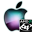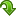Scilab Home Page | Wiki | Bug Tracker | Forge | Mailing List Archives | Scilab Online Help | File Exchange
ATOMS : Floating Point details

# Floating Point

Functions to manage floating point numbers
Details
Version
0.2.2
Author
Michael Baudin
Owner Organization
Consortium Scilab - Digiteo
Maintainers
John Gliksberg
Michael Baudin
Allan Cornet
Category
Dependencies
Creation Date
September 8, 2015
Source created on
Scilab 6.0.x
Binaries available on
Scilab 6.0.x:Windows 64-bitWindows 32-bitLinux 64-bitLinux 32-bitMacOSX
Install command
`--> atomsInstall("floatingpoint")`
Report a bugDescription
```            Purpose

The goal of this toolbox is to provide a collection of
algorithms for floating point number management.
This is more a learning tool than an operationnal component,
although it might complement some features which are not
provided by Scilab.

The flps_systemgui function allows to see the distribution
of floating point numbers graphically.
By varying the rounding mode, the precision and the logscale,
we can actually the distribution of a toy floating
point number. Adding or suppressing the denormals can be
instructive too.

The functions allow to compute automatically the
properties of the current Scilab system with
respect to the doubles. It is similar in spirit
to the number_properties functions, except that
our functions are based on macros and that
the returned values are made consistent
with the references cited in the bibliography.
Moreover, the flps_radix function returns
the current radix and allows to check that the
current rounding mode is round-to-nearest (which
is IEEE's default).

The functions allow to create virtual floating point
systems, which allows to see their discrete nature
in simplified examples. The rounding mode of
such a virtual floating point system can be configured
to one of the four mode from the IEEE standard.
This feature allows to see the effect of the rounding mode
on the distribution of floating point numbers. This is
not easy to see with a straightforward Scilab, since the
rounding mode is round-to-nearest, most of the time.

Features
-------

The following is a list of the current functions :

* Convert
* flps_Me2sm : Returns the (s,m) representation given (M,e).
* flps_double2hex : Converts a double into a hexadecimal string.
* flps_frombary : Returns the floating point number given its b-ary
decomposition.
* flps_hex2double : Converts a hexadecimal string into its double.
* flps_sme2M : Returns the integral significand from (s,m,e).
* flps_tobary : Returns the digits of the b-ary decomposition.
* Functions
* flps_chop : Round matrix elements to t significant binary places.
* flps_frexp : Returns the exponent and fraction.
* flps_minimumdecimalstr : Returns the minimum string for equality.
* flps_signbit : Returns the sign bit of x
* Number
* flps_number2hex : Converts a floating point number into a hexadecimal
string.
* flps_numbereval : Returns the value of the current floating point number.
* flps_numbergetclass : Returns the class of the number;
* flps_numberisfinite : Returns true if the number is finite.
* flps_numberisinf : Returns true if the number is an infinity.
* flps_numberisnan : Returns true if the number is a nan.
* flps_numberisnormal : Returns true if the number is bnormal.
* flps_numberissubnormal : Returns true if the number is subnormal.
* flps_numberiszero : Returns true if the number is zero.
* flps_numbernew : Returns a new floating point number.
* Properties
* flps_emax : Returns the maximum exponent and value before overflow.
* flps_emin : Returns the minimum exponent and value before underflow.
* flps_eps : Returns the machine epsilon and the precision for Scilab
doubles.
* flps_isIEEE : Returns true if the current system satisfies basic IEEE
requirements.
* flps_radix : Compute the radix used for Scilab doubles.
* System
* flps_systemall : Returns the list of floating point numbers of the given
floating point system.
* flps_systemgui : Plots all the numbers in the current floating point
system
* flps_systemnew : Returns a new floating point system.

Bibliography
------------

See DLAMCH for a comparison with Lapack's algorithms:
http://www.netlib.org/misc/dlamch.f

See number_properties in Scilab for a comparison :
modules/elementary_functions/sci_gateway/fortran/sci_f_number_properties.f

Author
------

Copyright (C) 2010 - DIGITEO - Michael Baudin
```Files (2)Source code archive
```
```OS-independent binary for Scilab 6.0.x
```Binary version
Automatically generated by the ATOMS compilation chain

```News (0)Comments (0)Leave a comment
You must register and log in before leaving a comment.Email notifications
Send me email when this toolbox has changes, new files or a new release.
You must register and log in before setting up notifications.